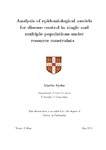## Analysis of epidemiological models for disease control in single and multiple populations under resource constraints

Vyska, Martin
##### DOIThesis
##### Abstract

Efficient management of epidemics is one of the primary motivations for computational modelling of disease dynamics. Examples range from reactive control measures, where the resources used to manage the epidemic in real time may be limited to prophylactic control measures such as deployment of genetically resistant plant varieties, which may lead to economic trade-offs. In such situations the question is how should resources for disease control be deployed to ensure the efficient management of the epidemic. Mathematical models are a powerful tool to investigate such questions since experiments are usually infeasible and the primary aim of this thesis is to study selected mathematical models of disease control to improve the current understanding of their behaviour. We initially analyse the dynamical behaviour that arises from incorporating an economic constraint into two simple, but widely used epidemic models with reactive control. Despite the selection of simple models, the addition of constrained control leads to mathematically rich dynamics, including the coexistence of multiple stable equilibria and stable limit cycles arising from global bifurcations. We use the analytical understanding obtained from the simple model to explore how to allocate a limited resource optimally between a number of separate populations that are exposed to an epidemic. Initially, we assume that the allocation is done at the beginning and cannot be changed later. We seek to answer the question of how the resource should be allocated efficiently to minimise the long-term number of infections. We show that the optimal allocation strategy can be approximated by a solution to a knapsack-type problem, that is the problem of how to select items of varying values and weights to maximise combined value without going over certain combined weight. The weights and values are given as functions of the population sizes, initial conditions, and the disease parameters. Later, we relax the assumptions to allow for reallocation and use the understanding of the dynamics gained from the simple models in the beginning to devise a new continuous time reallocation strategy, which outperforms previously considered approaches. In the final part of the thesis, we focus on plant disease and study a model of prophylactic control using a genetically resistant variety. We consider a trade-off where the genetic resistance carries with it a fitness penalty and therefore reduces yield. We identify the conditions on the parameters under which the resistant variety should be deployed and investigate how these change when the outbreak is uncertain. We show that deploying the resistant variety reduces the probability of an outbreak occurring and therefore can be optimal even when it would not be optimal to deploy it during the outbreak.